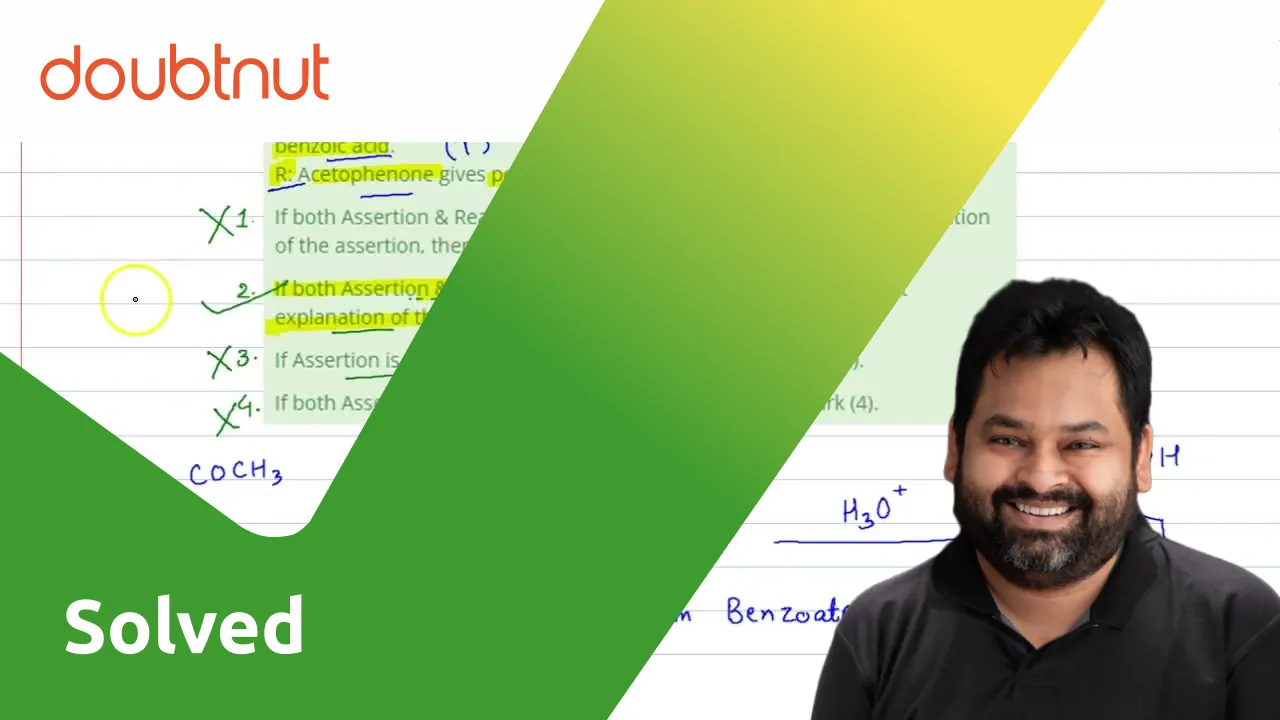Doubtnut App - Get 50 Lakh+ video solutions better than GoogleInstall Now

# In the following questions , a statement of assertion (A) is followed by a statement of reason (R). A: Acetophenone on reaction with NaOl followed by treatment with acid gives benzoic acid.R: Acetophenone gives positive iodoform test.

A

If both Assertion & Reason are true and the reason is the correct explanation of the assertion, then mark (1).

B

If both Assertion & Reason are true but the reason is not the correct explanation of the assertion, then mark (2).

C

If Assertion is true statement but Reason is false, then mark (3).

D

If both Assertion and Reason are false statements, then mark (4).

Video Solution